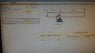# Gearbox torque calculation

Rajashekar
Hi team
In my one of the application I have to use the gear box to rotate 500 kg load
I have to use handwheel to rotate
I am using the formula
Torque= force x per. Distance x coefficient of friction
= 500x.7x.8 : 2800 Nm
Is it correct to calculate the torque

Regards
Raj

#### Attachments

•IMG-20160802-WA0005.jpeg
33.8 KB · Views: 658

benny_91
Hi team
In my one of the application I have to use the gear box to rotate 500 kg load
I have to use handwheel to rotate
I am using the formula
Torque= force x per. Distance x coefficient of friction
= 500x.7x.8 : 2800 Nm
Is it correct to calculate the torque

Regards
Raj
Your use of coefficient of friction seems incorrect. Moreover you have mentioned that a hand-wheel would be used for rotation but the figure shows something far different from a hand-wheel setup. If you can sketch the actual setup, take a pic of it and post it then it will be easier to answer your question.

Rajashekar
Your use of coefficient of friction seems incorrect. Moreover you have mentioned that a hand-wheel would be used for rotation but the figure shows something far different from a hand-wheel setup. If you can sketch the actual setup, take a pic of it and post it then it will be easier to answer your question.

Hi Benny